# On The Level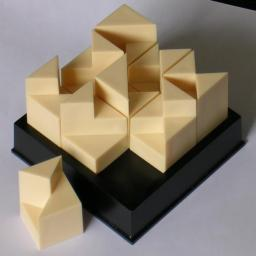On The Level is a tiling puzzle by Mag-Nif. It consists of 9 square tiles arranged in a 3×3 square. The tiles are split along their diagonals in 4 triangular sections. The tiles are not flat however, as each section is built up to one of three levels. The tiles could have been made simply with flat tiles with three colours, but this design is much more pleasing. There are nine possible distinct tiles for which all three height levels occur, and all are used in this puzzle.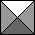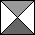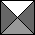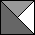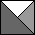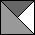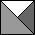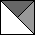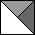The aim of the puzzle is to arrange the nine tiles in a square such that adjacent edges are of the same height, and also that the outside edges of the square match the height of the opposite edges.

## The number of positions:

There are 9 distinct tiles, so they have 9! arrangements. Each has 4 orientations, giving 49 orientations in total. This gives 9!·49 = 95,126,814,720 positions. This number is not a good representation of the difficulty since in practice you would place each tile to match the previous ones. This usually fixes the orientation of the placed tile, if it can be placed at all, so reducing the number of positions to check far below 9! = 362,880.
Given one solution, you can remove the top row and place it on the bottom (or vice versa) to get another solution. The same holds for the columns. Therefore the puzzle is always solvable regardless of where you place the first tile.

## Solution:

Here are three of the solutions: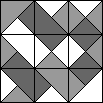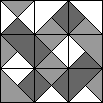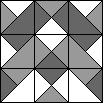As mentioned before, from one solution you can derive others by removing the top row and placing it on the bottom, or moving the left column to the right hand side. In this way nine related solutions are possible. Of course you can also rotate a solution or take its mirror image. Another way to generate further solutions is to exchange the heights. Since the tiles form a complete set, any permutation of the three heights is possible, generating up to six related solutions. Of course these solutions might be the same as others generated by shifting rows/columns and/or reflection/rotation. The third solution above is especially symmetric, giving rather few variations.

All the remaining solutions are variations of the following: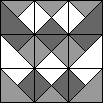This solution has the curious property that the top edge of each row is at the same height. This means that not only can variations be generated by the ways outlined before, each row can individually be shifted to the left or right.